Frequent Subtree Mining Algorithm for Ribonucleic Acid Topological Pattern

Frequent Subtree Mining Algorithm for Ribonucleic Acid Topological Pattern

Zhiqiang Li  Chengjie Xu   Chen Liu

School of Computer Science and Engineering, Yulin Normal University, Yulin 537000, China

School of Science, Hunan University of Technology, Zhuzhou 412007, China

Corresponding Author Email:
liuchen6949@163.com
Page:
75-80
|
DOI:
https://doi.org/10.18280/ria.330113
12 October 2018
|
Accepted:
28 January 2019
|
Published:
1 May 2019
| Citation

OPEN ACCESS

Abstract:

In recent years, with the demand for pattern information in the field of bioinformatics research, frequent subtree mining algorithms have become a research hotspot. In this paper, a fast and efficient mining algorithm based on frequent embedded subtree is proposed to solve the problem of large-scale of biological data and high sequence pattern similarity in the process of biological data mining. The algorithm uses a unique string coding method to represent the tree, and uses a scope-list for substring amplification and frequency testing. The pruning technique greatly compresses the search space and reduces the computational time. Compared with the classical pattern mining algorithm, the proposed algorithm improves the efficiency of mining greatly.

Keywords:

ribonucleic acid, frequent subtree, topological pattern, frequent pattern mining

1. Introduction

In recent years, a growing attention has been paid to the mining of frequent patterns in transaction databases, sequences, trees and graphs [1, 2]. The essence of frequent pattern mining is to discover useful correlations between patterns in numerous databases. Currently, frequent subtree mining is a research hotspot due to the increasing complexity of new structures and the rising demand for pattern information in bioinformatics.

In bioinformatics, frequent pattern mining of graphs and trees are critical to predicting and analyzing protein structure, as well as excavating compounds. Many ribonucleic acid (RNA) structures have been found and identified as tree structures. There is only one way to acquire information of a newly generated RNA structure: judge whether there is a common topology pattern between the new structure and known RNA structures. The judgement provides important reference for determining the molecular functions of new RNAs.

Many algorithms have emerged for the mining of frequent subtrees. Due to the influence of Apriori algorithm , most of the current subtree mining algorithms adopt a breadth-first search strategy. By this strategy, the common subtrees of all the trees in the dataset must be listed clearly. The common trees are enumerated in two common ways : the depth-first strategy of pattern growth and the breadth-first strategy of layer-by-layer mining.

The Tree Finder algorithm  is a typical method to mine unordered subtrees. However, the algorithm cannot mine out all frequent subtrees if different trees have the same tags or the support is very small. The popular algorithms for mining unordered direct subtrees include HybridTreeMiner, uNot, uFreqt, FreeTreeMiner and PathJoin [6-10]. If applied directly, these algorithms cannot achieve a high efficiency or make effective use biological features of deoxyribonucleic acid (DNA) or the data of protein sequence.

The inefficiency arises from the wide adoption of Apriori algorithm and its improved versions in frequent subtree algorithms. Relying on candidate set generation-screening, the original and improved Apriori algorithms only work efficiently in handling simple patterns. Facing a large candidate set, these algorithms will be very time-consuming, requiring multiple scans of the database.

The ineffective use of biological information is attributable to the limited ability of frequent pattern mining algorithms. These algorithms are only suitable for processing simple frequent sequence patterns and frequent items, but not capable of tackling complex biological data.

To solve the two problems, this paper proposes a frequent subtree mining algorithm based on biological data. The proposed algorithm describes the trees by a unique string encoding method, and uses the scope-list for subtree expansion and frequency testing. Experimental results show that the proposed algorithm greatly reduces the search space and shortens the runtime.

2. Basic Concepts and Problem Definition

2.1 Frequent subtree mining

Definition 1 (Subtree) Let T be a rooted tree, in which each node is denoted as x. Then, a subgraph derived from all the sub nodes of x is called a subtree of T, with x be its root.

Let |T| be the number of nodes of T, i.e. the size of the tree. Each node of the tree is given a serial number i(i=0…|T-1|) based on its position in the depth-first search. The node given the serial number i is denoted as $\mathbf{n}_{\mathbf{i}}$. Then, the items of a node can be allocated to a set L={0,1,2,3,…,m-1}, where m is the number of tags in the tree. For any node $\mathrm{v}_{\mathrm{i}} \in \mathrm{N}, \mathrm{L}\left(\mathrm{v}_{\mathrm{i}}\right)$ is the tag of node $\mathrm{V}_{\mathrm{I}}$, and $\mathrm{n}\left(\mathrm{v}_{\mathrm{i}}\right)$ is the serial number of node $\mathrm{v}_{\mathrm{i}}$. Each branch b consists of an ordered pair of nodes, where node $v_x$ is the parent of $v_y$. If it is not connected, the branch is called a subtree of T. In Figure 1, $S_1$, $S_2$ and $S_3$ are all subtrees of T.

1.jpg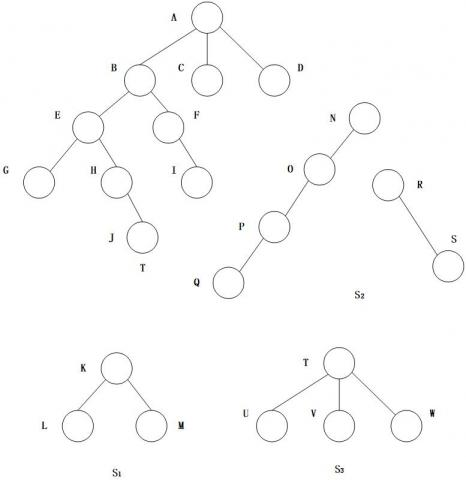Figure 1. A rooted tree and its subtrees

Definition $2\left(\text { Rooted subtree) Let } \mathrm{T}^{\prime}\left(V^{\prime}, \mathrm{E}^{\prime}, \Sigma^{\prime}, \mathrm{L}^{\prime}, r\right) \text { be a subtree of tree } \mathrm{T}(\mathrm{V}, \mathrm{E}, \Sigma, \mathrm{L}, r) \text { . If and only if, } V^{\prime} \in V, \mathrm{E}^{\prime} \in E,\right.$$\Sigma^{\prime} \in \Sigma$ and $\mathrm{L}^{\prime}, \in L, r$ is the root node of trees $\mathrm{T}^{\prime}$ and $\mathrm{T}$.

Definition 3 (Maximum frequent subtree and closed frequent subtree) For a tree data set $\mathrm{D}=\left\{T_{1}, T_{2}, \dots, T_{n}\right\}$, then a subtree T is the maximum frequent subtree in the data set, if T is frequent and any of its hyper trees is infrequent, and the subtree T is a closed frequent subtree, if T is frequent and greater than the support of any hyper tree.

Table 1. The tags and node fields of each node of tree T

 Tags Node fields L(A)=0 S(A)=[0, 6] L(B)=1 S(B)=[1, 5] L(D)=3 S(D)=[2, 4] L(F)=1 S(F)=[3, 3] L(G)=2 S(G)=[4, 4] L(E)=2 S(E)=[5, 5] L(C)=2 L(H)=1 S(C)=[6, 6] S(H)=[1, 4] L(I)=2 S(I)=[2, 2] L(J)=1 S(J)=[3, 2] L(K)=2 S(K)=[0, 2] L(L)=2 S(L)=[5, 1] L(M)=3 S(M)=[4, 3] L(N)=1 S(N)=[4, 3] L(O)=2 S(O)=[1, 1] L(P)=2 S(P)=[1, 2] L(Q)=3 S(Q)=[2, 3] L(R)=1 S(R)=[2,4] L(S)=2 S(S)=[3,1] L(T)=1 S(T)=[4,2] L(U)=2 S(U)=[3,2] L(V)=2 S(V)=[6,2] L(W)=2 S(W)=[6,3]

Table 1 lists the tags and node fields of each node of tree T in Figure 1. The set of nodes and the set of tagged nodes are respectively L={0,1,2,3} and N={A,B,C,D,E,F,G,H,I,J,K,L,M,N,O,P,Q,R,S,T,U,V,W}.

The serial number of each node in the tree of Figure 1 were determined by the sequence of the depth-first search (Table 2).

Table 2. The serial number of each node

 Node A B C D E F G Serial number 0 1 6 2 5 3 4

 Node A B C D E F G H I J Serial number 0 1 8 9 2 6 3 4 7 5

From Figure 1, it can be seen that L(A)=0, and the rightmost leaf node is D, then the root A domain s=[n(A), n(C)]=[0,9].

According to the above definitions, there must be fewer maximum frequent subtrees than closed frequent subtrees. Therefore, it is more efficient to mine the maximum frequent subtrees than the closed frequent subtrees, despite a slightly more information loss.

2.2 Subtree scalability check

In general, the tree structure can be represented by depth-first method or breadth-first method. Nevertheless, neither the depth-first method nor the breadth-first method can describe a tree structure alone without additional information.

The results of the two methods are known as the depth-first sequence and the breadth-first sequence, respectively. In the depth-first sequence, the nodes of a subtree are described as adjacent to each other; in the breadth-first sequence, the nodes of the same layer are illustrated as adjacent to each other.

Based on these two methods, this paper attempts to design a closed frequent subtree mining algorithm that identifies the subtree structure efficiently and completely. Since the subtree nodes of a column are not adjacent to each other, the depth-first method was applied several times to get all the nodes of each subtree. Then, the breadth-first sequence was obtained directly according to the hierarchical relationship between the nodes of the subtree. This hierarchical depth-first method can reflect the tree-shape data structure and pinpoint the nodes on subtrees of different root nodes.

Definition 4 (node level): Each tree can be defined by the level of its root node.

Definition 5 (node representation): Let R be a node on a tree, S be its parent and L be the level of the parent. Then, the node R can be expressed as <R, S, L>. If R is the root node, then the parent node and its level are both zeros.

Definition 6 (linear regular expression): For each tree, the depth-first sequence obtained by each node is a linear regular expression of the tree.

According to Definition 6, the tree in Figure 2(a) can be described as the sequence: <A, 0, 0>, <B, A, 1>, <D, B, 2>, <E, B, 2>, <C, A, 1>.

Based on the sequence, the three can be restored uniquely. For example, Figure 2(b) is the tree structure restored from the sequence <A, 0, 0>, <B, A, 1>, <D, B, 2>, <E, B, 2>, <C, A, 1>, <F, C, 2>.

2.jpgFigure 2. A tree and a restored tree

3. Frequent Subtree Mining Algorithm

Through the above analysis, a new algorithm was developed to mine frequent subtrees in biological data. In the algorithm, a scope-list is initialized based on the input tree database, and a string encoding method for these trees is adopted to create a string set. Specifically, a candidate set is generated from a node on the smallest subtree, and then expanded on each subtree by gradually adding the rightmost leaf node. This is to eliminate any redundant candidate subtree. During the subtree expansion, the support of each subtree was calculated to reflect the subtree frequency. To ensure the diversity of the candidate set, all infrequent subtrees are removed, leaving only the frequent ones.

The extension to the candidate subtree $T_i$ is realized by performing the rightmost expansion with the code string $\mathrm{C}\left(\mathrm{T}_{\mathrm{i}}\right)$ corresponding to that subtree. During the rightmost expansion of $\mathrm{C}\left(\mathrm{T}_{\mathrm{i}}\right)$, a new string C' is formed simply by adding a character after the string $\mathrm{C}\left(\mathrm{T}_{\mathrm{i}}\right)$. Since the algorithm only extends the scalable substring corresponding to the subtree, the resulting new string C' does not necessarily represent a subtree. Hence, the scalability of the new string must be verified. The scope-lists are created to extend all extensible subtrees. This process is repeated until all eligible subtrees have been generated. Finally, the acceptable frequent subtrees are identified through frequency testing.

During the computation, the search space is greatly compressed by the scalability check of subtrees and the use of scope-lists. This greatly enhances the overall performance of our algorithm.

Node insertion and deletion is the key to the compression of the search space. Node insertion should not undermine the position of the current node in the original tree, i.e. its relationship with other nodes in the sequence. For each compressed edge, a node is inserted into the compressed structure. Meanwhile, the corresponding edge in the original tree is hidden.

Node deletion is also indispensable to search space compression. To delete a node, a child node should be inserted to the position of the parent of the deleted node, and the information of the parent node of the deleted node should be updated accordingly. After the positions of all related nodes have been processed, the current node should be removed.

The overall framework of the proposed algorithm is as follows:

Algorithm 1 Frequent Subtree Mining Algorithm

Input: Tree database TDB, Minimum support min _sup;

Output: All frequent subtrees;

Scan (Edge a,min_sup){

child_node=a.second;

parent_node=a.first;

Insert Node (child_node, parent_node);

For each(n in child_node. children) {

}

DelNode (child_node);

If n<min_sup;

Return parent_node;

}

3.jpg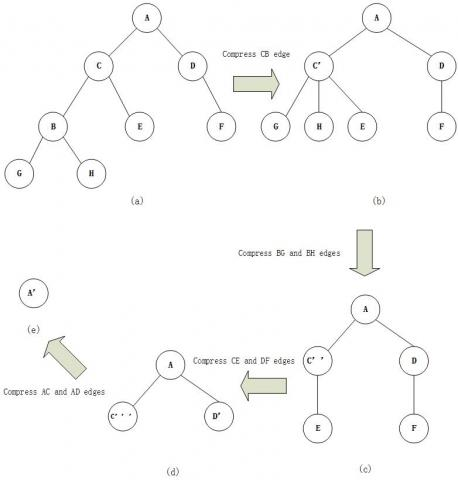Figure 3. Complete process of frequent subtree mining

Figure 3 details the entire process of frequent subtree mining for a tree structure ACBGHEDF. Firstly, the edge CB is compressed, with the sequence of node C’ being <C, A, 1>, <B, C, 2>. Then, edges BG and BH are compressed, with the sequence of node C’’ being <C, A, 1>, <B, C, 2>, <H, B, 3>, <G, B, 3>. After that, edges CE and DF are compressed, with the sequence of node C’’ being <C, A, 1>, <E, C, 2>, <B, C, 2>, <H, B, 3>, <G, B, 3>, and that of node D' being <D, A, 1>, <F, D, 2>. Finally, edges AC and AD are compressed, with the sequence of node A being <A, 0, 0>, <D, A, 1>, <F, D, 2>, <C, A, 1>, <E, C, 2>, <B, C, 2>, <H, B, 3>, <G, B, 3>. Through the above process, the original tree is fully compressed into a point. In each step, each edge being compressed can be regarded as a candidate frequent subtree, that generates frequent subtrees while compressing the space. The search efficiency is thus improved.

The obtained representation can restore the tree structure in a unique manner. As shown in Figure 4, the final tree sequence is <A,0,0>, <D,A,1>, <F,D,2>, <C,A,1>, <E,C,2>, <B,C,2>, <H,B,3>, <G,B,3>. Based on this sequence, the tree structure can be restored as follows:

Starting with the first node, the root node is <A, 0, 0>, the parent node is 0, and the level of the parent node is 0. Thus, node A is a root node. The next step is to search for the child node of A, i.e. the node whose parent node level is 1 below node A. Through the search, two sequences of children nodes can be identified, namely, <D, A, 1> and <C, A, 1>. This means D and C are the children of node A. The sequence between the two children is the subtree with D as the root node, and that between C and X is the subtree with C as the root node. Then, the tree structure can be restored recursively based on the two children sequences.

4.jpgFigure 4. Restoration of tree structure

4. Experiment and Results Analysis

4.1 Comparison of algorithm performance

The data on tree structure for our experiment are two popular datasets, namely, F5 and D10, which are manually generated based on real trees. The experiment aims to generate a parent tree with q tags and p nodes. The values of q and p were set to 5,000 and 200, respectively.

Firstly, the proposed algorithm was compared with two typical subtree mining algorithms (i.e. PatternMatcher (PM) algorithm  and TreeMiner (TM) algorithm ) in terms of runtime, as the data scale increased from t=100 to t=106, with the support remains at 0.5.

5.jpgFigure 5. Time variation of the number of trees

The results in Figure 5 show that the runtime of all three algorithms extended with the growing scale of data. However, when the data volume exceeded 100,000, the runtime of the PM algorithm rose sharply. This is mainly because the PM algorithm needs to scan the database repeatedly, and check the large candidate set via pattern matching. By contrast, both the TM and our algorithm consumed a much shorter time than the PM. In addition, the runtime of our algorithm increased slower than that of the TM, thanks to its superior pruning strategy. In summary, our algorithm outperformed the PM and the TM in an environment of massive data.

Next, the three algorithms were simulated again under different supports (from 0.1 to 1), while the input data scale was fixed on two scales (t=104 and t=105). This simulation attempts to compare the adaptability of each algorithm to support, which is generally negatively correlated with the runtime.

According to the runtime-support curves in Figures 6 and 7, the three algorithms consumed shorter runtimes with the increase of support, under whoever data scale. In the beginning, the runtime of the PM was much longer than that of the TM and that of our algorithm. Once the support reached 0.9, the PM saw greater decline in runtime than the TM and our algorithm. These results can be explained as follows:

6.jpgFigure 6. The relationship between the support and time (T=10,000)

In horizontal mining, the pruning operation can be performed in an Apriori manner. The specific strategy is to eliminate some infrequent subtrees through hierarchical search. When the support is approximately 1, most of the candidate modes will be removed. In practice, however, the user-defined support is generally smaller than 0.5, which is far below 1. When the support is less than 0.9, the runtime of the vertical mining algorithm TM and our algorithm is obviously shorter than the PM.

It can also be seen that the runtime of our algorithm under both data scales was little affected by the change of support, showing a relatively stable trend. Hence, our algorithm is more adaptable than the PM. Moreover, our algorithm consumed fewer time than the TM, a sign of its excellent efficiency.

7.jpg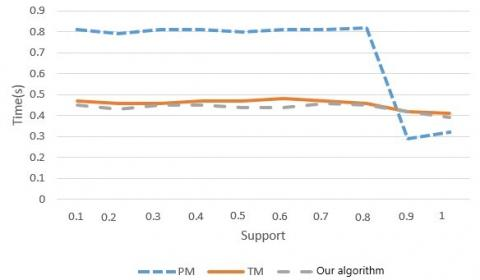Figure 7. The relationship between the support and time (T=100,000)

4.2 RNA experiments

From the RNase P Database , some RNA molecular structures were extracted to develop a tree model. Then, the common tree topology of 30 trees was mined by our algorithm.

Figure 8 shows the relationship between support and the runtime of our algorithm. With the decline in support, the number of acceptable frequent patterns being mined gradually increased, and the runtime was also extended.

8.jpgFigure 8. Relationship between runtime and support

Figure 9 shows the variation in the number of frequent patterns mined by our algorithm under different supports. Obviously, the number of frequent patterns being mined gradually increased with the decrease of support. However, when the support approximated zero, the number of frequent patterns being mined was not significantly affected. The growth rate of frequent patterns was relatively stable, when the support varied in [0.3, 0.5]. In this case, the frequent patterns obtained by our algorithm are typical representations of the selected 30 tree structures.

9.jpg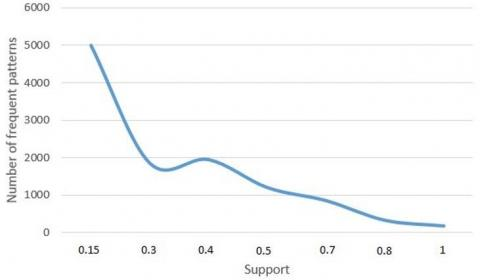Figure 9. Relatinship between the number of frequent patterns and support

Figure 10 shows a subtree mined at least 9 times by the proposed algorithm in 30 trees, which was not found by other algorithms. Therefore, compared with other direct subtree mining algorithms, the proposed algorithm can mine more “hidden” information for the mining of RNA subtrees, and at the same time, it makes the mined results more biologically meaningful.

10.jpg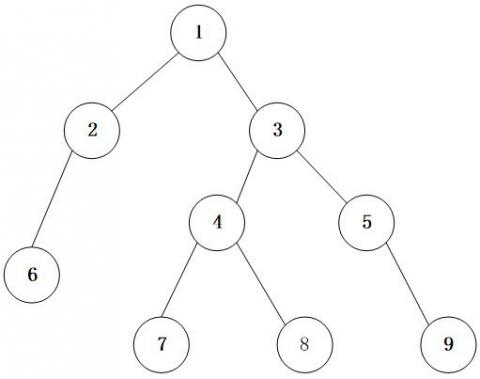Figure 10. A subtree that is mined

5. Conclusion

This paper designs a fast and effective frequent subtree mining algorithm for biological data mining. The algorithm adopts a tree structure compression method, which is inspired by the representation of tree structure. The compression method optimizes the mining of frequent subtrees, and records the intermediate results in the mining process. In addition, our algorithm compresses multiple edges according to the current frequency at each time, and then judges whether the compressed subtree is a frequent pattern. Therefore, our algorithm can process multiple nodes and edges in each round, and achieve an efficiency about 118% of the traditional method. If the compressed subtree is not frequent, the frequent subtrees are mined on smaller datasets by prefix matching, such that our algorithm can mine all frequent subtrees. Finally, the feasibility and efficiency of the algorithm were verified through experiments on real datasets.

Acknowledgements

This work is supported by the National Natural Science Foundation of China (No.61841603), Guangxi Natural Science Foundation (No.2018JJA170050, No.2014GXNSFBA118268), Science and Technology Research Foundation of Guangxi Universities (201204LX339).

References

 Arnold, R., Goldenberg, F., Mewes, H.W. (2014). SIMAP-the database of all-against-all protein sequence similarities and annotations with new interfaces and increased coverage. Nucleic Acids Research, 42(1): 279-284. https://doi.org/10.1093/nar/gkt970

 Slater, G.S.C., Ewan, B. (2005). Automated generation of heuristics for biological sequence comparison. Bmc Bioinformatics, 6(1): 1-11. https://doi.org/10.1186/1471-2105-6-31

 Toivonen, H. (2011). Apriori Algorithm. Encyclopedia of Machine Learning, 39-40. https://doi.org/10.1007/978-0-387-30164-8_27

 Tan, Z., Sharma, G., Mathews, D.H. (2017). Modeling RNA secondary structure with sequence comparison and experimental mapping data. Biophysical Journal, 113(2): 330-341. https://doi.org/10.1016/j.bpj.2017.06.039

 Termier, A., Rousset, M.C. (2002). TreeFinder: A first step towards XML data mining. IEEE International Conference on Data Mining, IEEE Computer Society, 450-458. https://doi.org/10.1109/ICDM.2002.1183987

 Zaki, M.J. (2004). TreeMiner: An efficient algorithm for mining embedded ordered frequent trees. Advanced Information & Knowledge Processing, 123-151. https://doi.org/10.1007/1-84628-284-5_5

 Pasquier, C., Sanhes, J., Flouvat, F. (2016). Frequent pattern mining in attributed trees: algorithms and applications. Knowledge and Information Systems, 46(3): 491-514. https://doi.org/10.1007/s10115-015-0831-x

 Jiang, C., Coenen, F., Zito, M. (2013). A survey of frequent subgraph mining algorithms. The Knowledge Engineering Review, 28(1): 75-105. https://doi.org/10.1017/s0269888912000331

 Asai, T., Arimura, H., Uno, T. (2003). Discovering frequent substructures in large unordered trees. Lnai, 2843: 47-61. https://doi.org/10.1007/978-3-540-39644-4_6

 Babbar, A., Singh, A., Singh, D. (2014). A survey on problems and solutions of frequent pattern mining with the use of pre-processing techniques. International Journal of Computer Applications, 95(1): 23-28. https://doi.org/10.5120/16559-4125

 Okosun, J., Csaba, B., Wang, J. (2014). Integrated genomic analysis identifies recurrent mutations and evolution patterns driving the initiation and progression of follicular lymphoma. Nature Genetics, 46(2): 176-181. https://doi.org/10.1038/ng.2856

 Zhang, S., Du, Z., Wang, J.T. (2015). New techniques for mining frequent patterns in unordered trees. IEEE Transactions on Cybernetics, 45(6): 1113-1125. https://doi.org/10.1109/tcyb.2014.2345579

 Liu, M.H., Yuan, Y., Reddy, R. (1994). Human RNaseP RNA and nucleolar 7-2 RNA share conserved 'To' antigen-binding domains. Molecular & Cellular Biochemistry, 130(1): 75-82. https://doi.org/10.1007/BF01084270